# Maharashtra Board 8th Class Maths Practice Set 8.2 Solutions Chapter 8 Quadrilateral: Constructions and Types

Balbharti Maharashtra State Board Class 8 Maths Solutions covers the Practice Set 8.2 8th Std Maths Answers Solutions Chapter 8 Quadrilateral: Constructions and Types.

## Practice Set 8.2 8th Std Maths Answers Chapter 8 Quadrilateral: Constructions and Types

Question 1.
Draw a rectangle ABCD such that l(AB) = 6.0 cm and l(BC) = 4.5 cm.
Solution: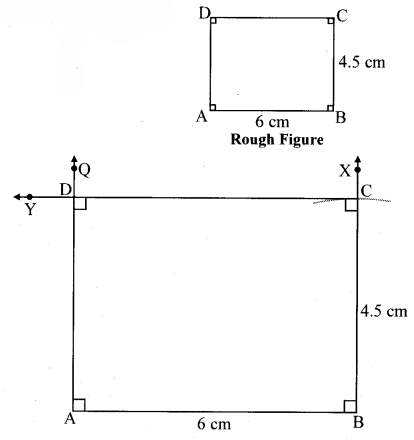Question 2.
Draw a square WXYZ with side 5.2 cm.
Solution: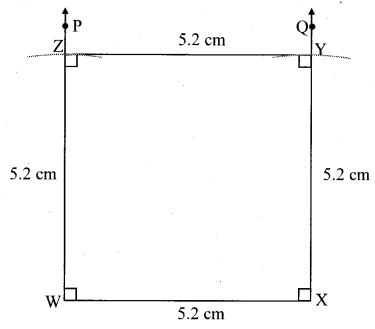Question 3.
Draw a rhombus KLMN such that its side is 4 cm and m∠K = 75°.
Solution: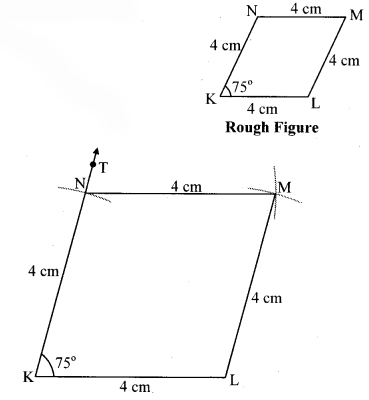Question 4.
If diagonal of a rectangle is 26 cm and one side is 24 cm, find the other side.
Solution: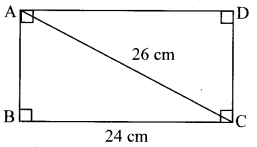Let ₹ABCD be the rectangle.
l(BC) = 24cm, l(AC) = 26cm
In ∆ABC,
m∠ABC = 90° …[Angle of a rectangle]
∴[l(AC)]² = [l(AB)]2 + [l(BC)]²
…[Pythagoras theorem]
∴ (26 )² = [l(AB)]² + (24)²
∴(26)² – (24)² = [l(AB)]²
∴(26 + 24) (26 – 24) = [l(AB)]²
…[∵ a² – b² = (a + b)(a – b)]
∴50 x 2 = [l(AB)]²
∴100 = [l(AB)]²
i.e. [l(AB)]² = 100
∴l(AB) = √100
…[Taking square root of both sides]
∴l(AB) =10 cm
∴The length of the other side is 10 cm.

Question 5.
Lengths of diagonals of a rhombus ABCD are 16 cm and 12 cm. Find the side and perimeter of the rhombus.
Solution:
In rhombus ABCD,l(AC) = 16 cm and l(BD) = 12 cm.
Let the diagonals of rhombus ABCD intersect at point O.
l(AO) = $$\frac { 1 }{ 2 }$$ l(AC)
…[Diagonals of a rhombus bisect each other]
∴l(AO) = $$\frac { 1 }{ 2 }$$ × 16
∴l(AO) = 8 cm
Also, l(DO) = $$\frac { 1 }{ 2 }$$ l(BD)
…[Diagonals of a rhombus bisect each other]
∴l(DO) = $$\frac { 1 }{ 2 }$$ × 12
∴l(DO) = 6 cm
In ∆DOA,
m∠DOA = 90°
..[Diagonals of a rhombus are perpendicular to each other]
…[Pythagoras theorem]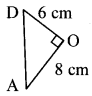= (8)² + (6)²
= 64 + 36
… [Taking square root of both sides]
∴l(AB) = l(BC) = l(CD) = l(AD) = 10 cm
…[Sides of a rhombus are congruent]
Perimeter of rhombus ABCD
= l(AB) + l(BC) + l(CD) + l(AD)
= 10+10+10+10
= 40 cm
∴The side and perimeter of the rhombus are 10 cm and 40 cm respectively.

Question 6.
Find the length of diagonal of a square with side 8 cm.
Solution:
Let ₹XYWZ be the square of side 8cm.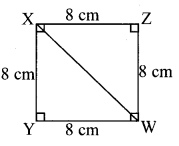seg XW is a diagonal.
In ∆ XYW,
m∠XYW = 90°
… [Angle of a square]
∴ [l(XW)]² = [l(XY)]² + [l(YW)]²
…[Pythagoras theorem]
= (8)² + (8)²
= 64 + 64
∴ [l(XW)]² = 128
∴ l(XW) = √128
…[Taking square root of both sides]
= √64 × 2
= 8 √2 cm
∴ The length of the diagonal of the square is 8 √2 cm.

Question 7.
Measure of one angle of a rhombus is 50°, find the measures of remaining three angles.
Solution:
Let ₹ABCD be the rhombus.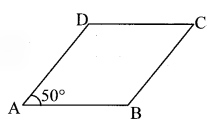m∠A = 50°
m∠C = m∠A
….[Opposite angles of a rhombus are congruent]
∴ m∠C = 50°
Also, m∠D = m∠B …(i)
….[Opposite angles of a rhombus are congruent]
In ₹ABCD,
m∠A + m∠B + m∠C + m∠D = 360°
….[Sum of the measures of the angles of a quadrilateral is 360°]
∴ 50° + m∠B + 50° + m∠D = 360°
∴ m∠B + m∠D + 100° = 360°
∴ m∠B + m∠D = 360° – 100°
∴ m∠B + m∠B = 260° …[From (i)]
∴ 2m∠B = 260°
∴ m∠B = $$\frac { 260 }{ 2 }$$
∴ m∠B = 130°
∴ m∠D = m∠B = 130° …[From (i)]
∴ The measures of the remaining angles of the rhombus are 130°, 50° and 130°.

Maharashtra Board Class 8 Maths Chapter 8 Quadrilateral: Constructions and Types Practice Set 8.2 Intext Questions and Activities

Question 1.
Construct a rectangle PQRS by taking two convenient adjacent sides. Name the point of intersection of diagonals as T. Using divider and ruler, measure the following lengths.
i. lengths of opposite sides, seg QR and seg PS.
ii. lengths of seg PQ and seg SR.
iii. lengths of diagonals PR and QS.
iv. lengths of seg PT and seg TR, which are parts of the diagonal PR.
v. lengths of seg QT and seg TS, which are parts of the diagonal QS.
Observe the measures. Discuss about the measures obtained by your classmates. (Textbook pg. no. 44)
Solution:
Draw a rectangle PQRS such that, l(PQ) = 3 cm and l(QR) = 4 cm.Steps of construction:
i. As shown in the rough figure, draw seg QR of length 4 cm.
ii. Placing the centre of the protractor at point Q, draw ray QW making an angle of 90° with seg QR.
iii. By taking a distance of 3 cm on the compass and placing it at point Q, draw an arc on ray QW. Name the point as P.
iv. Draw ray PV and ray RU making an angle of 90° with seg PQ and seg QR respectively.
v. Name the point of intersection of ray PV and ray RU as S.
₹PQRS is the required rectangle.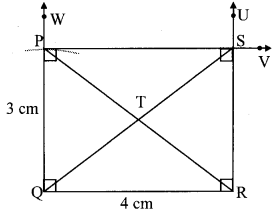From the figure,
i. l(QR) = l(PS) = 4 cm
ii. l(PQ) = l(SR) = 3 cm
iii. l(PR) = l(QS) = 5 cm
iv. l(PT) = l(TR) = 2.5 cm
v. l(QT) = l(TS) = 2.5 cm

From the above measures, we can say that for any rectangle,
i. Opposite sides are congruent.
ii. Diagonals are congruent.
iii. Diagonals bisect each other.

Question 2.
Draw a square by taking convenient length of side. Name the point of intersection of its diagonals as E. Using the apparatus in a compass box, measure the following lengths.
i. lengths of diagonal AC and diagonal BD.
ii. lengths of two parts of each diagonal made by point E.
iii. all the angles made at the point E.
iv. parts of each angle of the square made by each diagonal, (e.g. ∠ADB and ∠CDB).
Observe the measures. Also observe the measures obtained by your classmates and discuss about them. (Textbook pg. no. 44)
Solution:
Draw a square ABCD such that its side is 5cm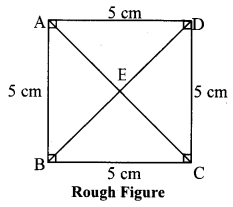Steps of construction:
i. As shown in the rough figure, draw seg BC of length 5 cm.
ii. Placing the centre of the protractor at point B, draw ray BP making an angle of 90° with seg BC.
iii. By taking a distance of 5 cm on the compass and placing it at point B, draw an arc on ray BP. Name the point as A.
iv. Placing the centre of the protractor at point C, draw ray CQ making an angle of 90° with seg BC.
v. By taking a distance of 5 cm on the compass and placing it at point C, draw an arc on ray CQ. Name the point as D.
₹ABCD is the required square.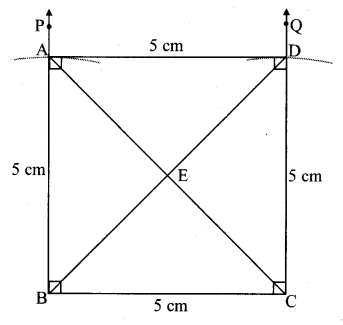From the figure,
i. l(AC) = l(BD) ≅ 7cm
ii. l(AE) = l(EC) ≅ 3.5cm,
l(BE) = l(ED) ≅ 3.5cm
iii. m∠AED = m∠BEC = m∠CED = m∠BEA = 90°
iv. Angles made by diagonal AC:
m∠BAC = m∠DAC = 45°
m∠BCA = m∠DCA = 45°
m∠ABD = m∠CBD = 45°

From the above measures, we can say that for any square,
i. Diagonals are congruent.
ii. Diagonals bisect each other.
iii. Diagonals are perpendicular to each other.
iv. Diagonals bisect the opposite angles.

Question 3.
Draw a rhombus EFGH by taking convenient length of side and convenient measure of an angle.
Draw its diagonals and name their point of Intersection as M.
i. Measure the opposite angles of the quadrilateral and angles at the point M.
ii. Measure the two parts of every angle made by the diagonal.
iii. Measure the lengths of both diagonals. Measure the two parts of diagonals made by point M.
Observe the measures. Also observe the measures obtained by your classmates and discuss about them. (Textbook pg. no. 45)
Solution:
Draw a rhombus EFGH such that its side is 5 cm and m∠F = 60°.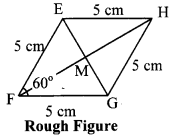Steps of construction:
i. As shown in the rough figure, draw seg FG of length 5 cm.
ii. Placing the centre of the protractor at point F, draw ray FX making an angle 60° with seg FG.
iii. By taking a distance of 5 cm on the compass and placing it at point F, draw an arc on ray FX. Name the point as E.
iv. By taking a distance of 5 cm on the compass and placing it at point E and point G, draw arcs. Name the point of intersection of arcs as H. ₹EFGH is the required rhombus.From the figure,
i. Opposite angles:
m∠EFG = m∠GHE = 60°,
m∠FEH = m∠HGF = 120°
Angles at the point M:
m∠EMF = m∠FMG = m∠GMH = m∠HME = 90°

ii. Angles made by diagonal FH:
m∠EFH = m∠GFH = 30° m∠EHF = m∠GHF = 30°